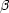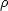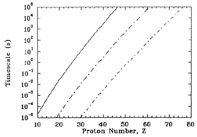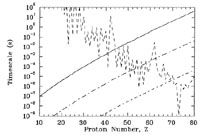Annu. Rev. Astron. Astrophys. 1994. 32: 153-90 Copyright © 1994 by Annual Reviews. All rights reserved

### 5. THE p-PROCESS

....and the elements shall melt with fervent heat

II Peter 3:10

We turn finally to the p-nuclei. These are the 35 nuclei bypassed by the r- and s-processes. As we see from Figure 1, except for the light p-nuclei (92Mo, 94Mo, 96Ru, 98Ru), the abundances of p-nuclei are considerably less than those of their r- and s-nuclei counterparts. Furthermore, the p-process abundance distribution shows interesting structure with peaks at 92Mo and 144Sm. These are important clues for determining where the p-process occurs.

It is probably wrong to think that the p-process occurs in a single site. We can imagine many astrophysical settings where conditions are right to modify a pre-existing supply of r- and s-nuclei to form p-nuclei. The relevant question is really what site contributes the bulk of the p-nuclei. For more details on the p-process, the reader should consult the excellent review by Lambert (1992).

It is impossible to produce p-nuclei by neutron capture. How then can nature make these nuclei? The first possibility that suggests itself is proton capture. It may be that in the course of the evolution of some system striving to reach NSE, protons are liberated which can capture on pre-existing seed nuclei to make p-nuclei. Alternatively it may be that in a freezeout from proton-rich NSE, free protons could capture on seed iron-group nuclei.+ reactions could allow further capture of protons to higher mass.

We can explore the conditions required for such p-processes with the help of Figures 9 and 10. Figure 9 shows the timescale for capture of a proton [a (p,) reaction] by the most proton-rich beta-stable isotope of each element at the fixed temperature of T9 = 1 for different values of the proton mass densityYp. The timescales for a proton-disintegration (, p) reaction or for a neutron-disintegration (, n) reaction for these isotopes are all greater than 1010 seconds. Note that for a site with a proton mass densityYp of 1 g cm-3, it would take 92Mo (Z = 42) about 104 s to capture a proton. Capture of protons on higher-charge isotopes would take even longer. The timescale for proton capture decreases if the setting has a higher density of protons available. For example, forYp = 103 g cm-3, the timescale for capture of protons on 92Mo would be about 10s while forYp = 106 g cm-3 it would be about 10-2 s. If an astrophysical site could maintain a mass density in protons of 106 g cm-3 for 105 s at T9 = 1, the proton-rich isotopes of all elements up to platinum (Z = 78) could capture a proton. The question for finding the p-process site is whether such conditions are possible. It is unlikely.Figure 9. Timescales for proton capture on the most proton-rich isotope of each element at the fixed temperature of T9 = 1. The curves are for mass densities in protons ofYp = 1 g cm-3 (solid curve), 103 g cm-3 (long dashed-dotted curve), and 106 g cm-3 (short dashed-Soiled curve). The rates are computed from expressions in Woosley et al (1975).Figure 10. Same as Figure 9 but for T9 = 3. The short-dashed jagged curve shows the timescale for (, n) reactions on these nuclei. The (, n) rates are computed from neutron-capture cross sections in Woosley & Hoffman or Cowan et al (1991) and neutron-separation energies are derived from Möller & Nix (1988).

The proton-capture rates also increase with increasing temperature because the reactants have a higher relative kinetic energy compared to the Coulomb barrier than at lower temperature. This leads us to ask what happens to the timescales if we increase the temperature. We see the effect in Figure 10 for T9 = 3. The timescale for proton capture does indeed decrease, but so does the timescale for a (, n) reaction. ForYp = 1 g cm-3, the timescale for a (, n) reaction is less than that for a (p,) reaction at Z40. This means that it is more likely under these conditions for a proton-rich nucleus to suffer a (, n) reaction than to capture a proton. Of course this makes sense from our discussion in Section 2. If the system is evolving towards NSE, nuclei more massive than the nucleus with the highest binding energy per nucleon will tend to disintegrate nucleons to increase the number of macroscopic states available to the system.

For higher proton mass densities, the (, n) reactions do not dominate the proton captures until higher nuclear charge: Z40 - 50 forYp = 103 g cm3 and Z70 forYp = 106 g cm-3. One might imagine that the system could then produce p-nuclei at least up to ytterbium (Z = 70) under such high temperature and high density conditions. Such conditions are extremely difficult for nature to achieve, however. The dilemma for making p-nuclei is clear. If nature is to make these nuclei by proton capture at low temperature where the flow will not be impeded by disintegrations, a large supply of protons must be available for a long time. If the proton capture process is to occur at higher temperature where the capture timescales are shorter, disintegration reactions will dominate the flow and prevent capture to higher mass.

The escape from this dilemma is the realization that proton captures need not make most of the p-nuclei. The various disintegration reactions do the job. In particular, if pre-existing r- and s-nuclei are exposed to high temperature, nuclear reactions will occur and tend to drive the abundances toward NSE. The first reactions to occur are the (, n) reactions which produce quite proton-rich nuclei. Once the nuclei become sufficiently proton rich, they then begin a (, p) and (,) cascade. In this way the nuclei "melt"towards iron. If the high temperature drops off quickly enough, the system does not reach NSE and the melting will be incomplete, leaving an abundance of proton-rich heavy nuclei - the p-nuclei. Where the disintegration flow crosses the N = 50 and N = 82 closed neutron shells, the disintegration timescales become large because of the particularly strong binding energies. This is apparent in Figure 10 as the peaks in the (, n) timescales at the closed shell nuclei 92Mo (Z = 42) and 144Sm (Z = 62). Because of the long disintegration timescales, abundances build up at these nuclei. In this way we can explain the peaks in the solar system's p-process abundance distribution. As a final note, the extremely short (, n) timescale for 180Ta (Z = 73 in Figure 10) explains why this fragile species is the rarest stable isotope in nature.